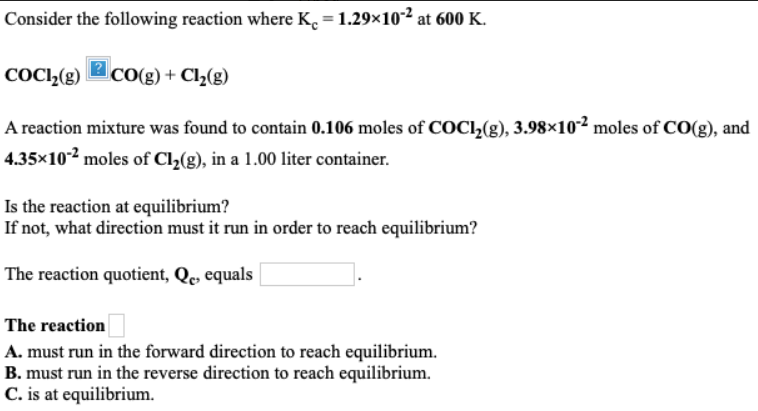# Consider the following reaction where Kc = 1.29 x 10^-2 at 600 K. COCl2(g) -> CO(g) + Cl2(g) A reaction mixture was found to contain 0.106 moles of COCl2(g), 3.98 x 10^-2 moles of CO(g), and 4.35 x 10^-2 moles of Cl2(g), in a 1.00 liter container. Is the reaction at equilibrium? If not, what direction must it run in order to reach equilibrium? The reaction quotient, Qc, equals ___. The reaction a. must run in the forward direction to reach equilibrium. b. must run in the reverse direction to reach equilibrium. c. is at equilibrium.